# A Description of R Package ‘dhh’

## Introduction

The name of this R package “dhh” can be understood as “Distributions with Heavy Heads”. The probability density function of the heavy-headed distribution is $f(x) = \frac{\alpha (b-a)^{-\alpha}}{(x-a)^{1-\alpha}}I\{a<x<b\},$ with parameters $$a<b$$ and $$\alpha>0$$. For the motivation and derivation of this distribution, please see the references.

This package contains four functions on a heavy-headed distribution:

• dhh: the probability density function
• phh: the cumulative distribution function (CDF)
• qhh: the quantile function
• rhh: the random variables

Next, I will introduce these functions with examples.

To use this library, we can first install it and then use the following command.

library(dhh)

## On dhh

This function dhh(x, a, b, alpha) gives the values of the density of the heavy-headed distribution.

The default values of a, b and alpha are 0, 1 and 0.1, respectively. Then, the following two commands give the same result, the value of the density at 0.5.

dhh(0.5)
#>  0.1866066
dhh(0.5, 0, 1, 0.1)
#>  0.1866066

The argument x can be a vector.

dhh(c(0.5, 0.7))
#>  0.1866066 0.1378516

We can take a look at the plots of some density functions.

curve(dhh(x, a=0, b=1, alpha=0.1), -1, 2)
curve(dhh(x, a=0, b=10, alpha=0.1), -1, 11)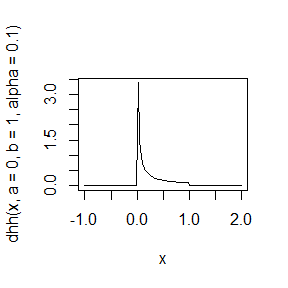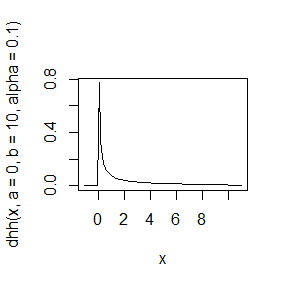## On phh

This function phh(x, a, b, alpha) gives the values of the CDF of the heavy-headed distribution.

The default values of a, b and alpha are 0, 1 and 0.1, respectively. Then, the following two commands give the same result, the value of the density at 0.5.

phh(0.5)
#>  0.933033
phh(0.5, 0, 1, 0.1)
#>  0.933033

The argument x can be a vector.

phh(c(0.5, 0.7))
#>  0.9330330 0.9649611

We can take a look at the plots of some CDF’s.

curve(phh(x, a=0, b=1, alpha=0.1), -1, 2)
curve(phh(x, a=0, b=10, alpha=0.1), -1, 11)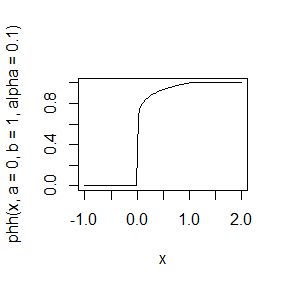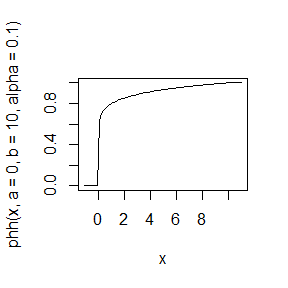## On qhh

This function qhh(p, a, b, alpha) gives the quantiles of the heavy-headed distribution.

The default values of a, b and alpha are 0, 1 and 0.1, respectively. Then, the following two commands give the same result, the value of the density at 0.5.

qhh(0.9)
#>  0.3486784
qhh(0.9, a=0, b=1, alpha=0.1)
#>  0.3486784

The argument p can be a vector.

phh(qhh((1:9)/10))
#>  0.1 0.2 0.3 0.4 0.5 0.6 0.7 0.8 0.9

We can take a look at the plots of some quantile functions.

curve(qhh(x, 0, 1, 0.1), from = 0.1, to = 0.9)
curve(qhh(x, a=10, b=100, alpha = 0.1), from = 0.1, to = 0.9)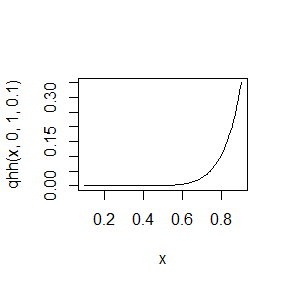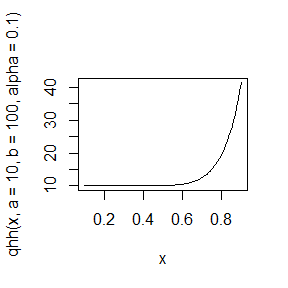## On rhh

This function rhh(n, a, b, alpha1) generate $$n$$ i.i.d. random variables following the heavy-headed distribution.

The default values of a, b and alpha are 0, 1 and 0.1, respectively. Then, the following two commands are the same.

rhh(5)
#>  1.252758e-04 4.515955e-03 1.327694e-02 1.447834e-13 1.917654e-02
rhh(5, a = 0, b = 1, alpha = 0.1)
#>  8.962788e-01 1.679130e-01 1.665611e-06 1.211562e-04 3.247341e-01

We can generate $$10000$$ i.i.d. random variables and plot the histogram. Then we can add the density plot.

hist(rhh(10000), freq=FALSE)
curve(dhh, add = TRUE, col = 2)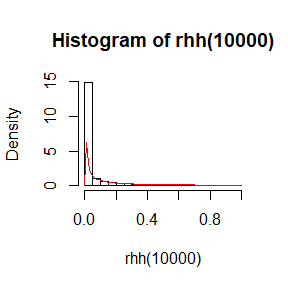We can also check the fact that the density goes to infinity as a goes to a.

dhh(c(0.1, 0.01, 0.001, 0.0001, 0.00001))
#>     0.7943282    6.3095734   50.1187234  398.1071706 3162.2776602